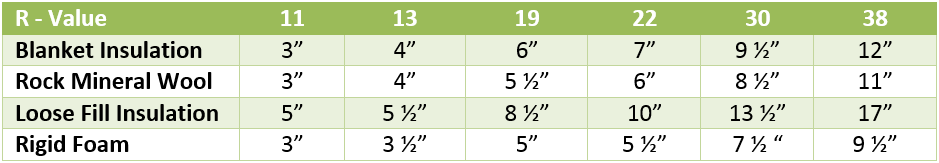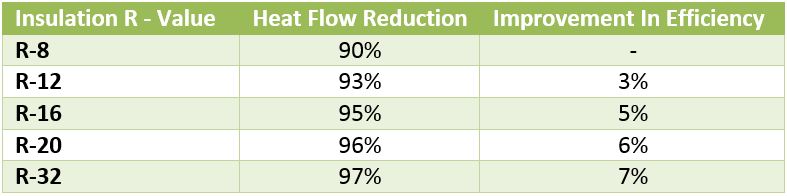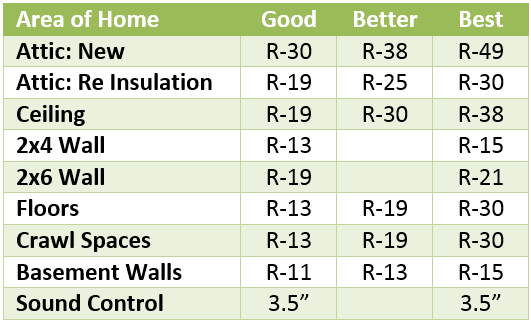Thermal Values of Insulation | The Renewable Energy Hub

# Thermal Values of Insulation

## R-Value

An R–Value rating is given to insulation in order to measure its thermal resistance with the standard measurement given in square metre kelvin per watt. It measures the temperature variation over insulation and also the amount of heat transferred through the insulating material. The higher the calculated number value the more effective the insulating material will be at preventing heat transfer. The R-values primary measure is the insulation materials ability to prevent or reduce heat flow.

 Thickness λ

## U-Value

Insulation can also be ranked using a U-value which calculates a materials insulating effectiveness in watts. A lower U value generally indicates that the material is a more effective insulator. U values are useful for calculating a building elements overall insulating effectiveness rather than just measuring the effectiveness of a specific material.

U-Values are calculated as the reciprocal of the sum of R values, i.e.

 1 Total sum of R values

So the lower figure reached in calculation, the better the insulation value of the structure

## Lambda (λ) Value (thermal conductivity)

Lambda value λ(w/mK)  is a measure of the rate at which heat passes through a material. The units are watts per unit thickness per degree temperature difference across that unit thickness. Examples include Glass mineral wool Loft roll at 0.044w/mk, and Celotex GA4000 PIR board at 0.022w/mk.

The lower the value, the better the insulating capacity of the product (for a given thickness).

## K-Value

Another measure of the effectiveness of insulation is the K-value which is used to measure the ability of the insulation material to conduct heat. Specifically, it is the measure of the amount of heat, in BTUs per hour that will be transmitted through one square foot of material that is one inch thick to cause a temperature change of one degree Fahrenheit from one side of the material to the other. A lower K value would indicate that the material is a good insulator. Because the numbers will vary between different products the EU has a 90/90 rule which means that 90% of the insulating product will conform to the K value with a confidence level of 90%.Press Esc to close
Press Esc to close